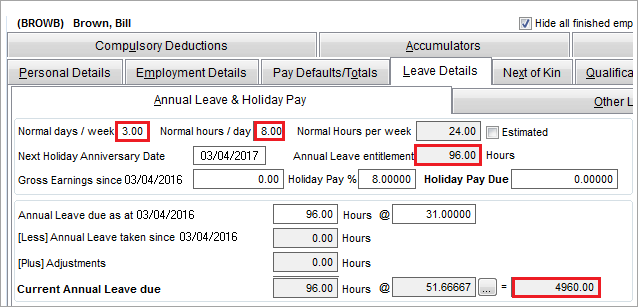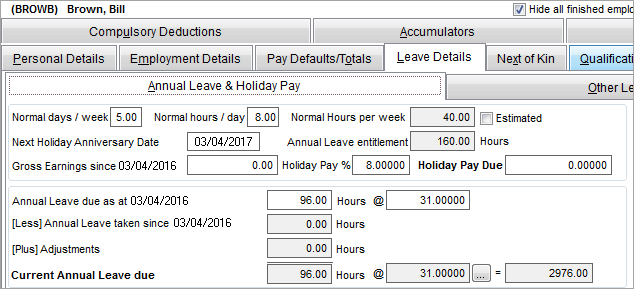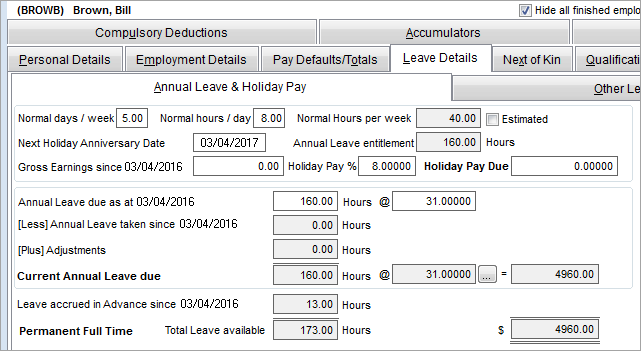##### Child pages
• Increasing an employee's Normal Hours per week

When you increase an employee's Normal Hours per week, their annual leave calculation rate will decrease but the allocation of annual leave will remain the same. This means the employee will appear to be owed less annual leave than they should have.

This support note explains how to increase an employee's Normal Hours per week, then how to correct their Current Annual Leave Due. If you're increasing a pay rate during a pay period, see Increasing an employee's pay rate part way through a pay period.

The example we'll use is Bill who is increasing his work hours from 24 to 40 hours per week.

Before you begin

Print the affected employee's accrued leave report for your records prior to making any adjustments. This report can be accessed by going to Reports > Employee Reports > Employee Holidays.

To increase an employee's Normal Hours per week
1. Go to the Maintenance command centre and click Maintain Employees.
2. Select the applicable employee.
3. Click the Leave Details tab.
4. Enter the required values in the Normal days/week and Normal hours/day fields. This will adjust their Normal Hours per week.

The Normal Hours per week setting is used:

• to allocate the annual leave entitlement for the year. For example an employee working 40 normal hours per week is allocated 160 hours leave at their anniversary date (4 weeks X 40 hours per week=160 hours).
• as a divisor for the calculation of the annual leave rate. The Average Weekly Earnings and the Ordinary Weekly Pay are divided by the Normal Hours per week to calculate the annual leave rate.

## What is the effect of increasing the Normal Hours per week?

As shown below, Bill currently works 8 hours a day, 3 days a week, totalling 24 hours a week. This gives Bill an annual leave allocation balance of 96 hours a year. His current annual leave due rate is \$51.66 which equates to \$4960 per year (\$51.66 x 96 hours).Here's what happens when we increase Bill's days per week from 3 to 5:This increases Bill's Annual Leave Entitlement to 160 hours a year, and decreases his rate to \$31, which is correct. However if no other changes are made, this makes the Current Annual Leave Due \$2976 (\$31 x 96 hours), a decrease of \$1984, which is incorrect.

The next task explains how to correct the Current Annual Leave Due.

To correct the Current Annual Leave Due

To correct the Current Annual Leave Due, you'll need to make an adjustment to the number of hours in the Annual Leave due as at field. In Bill's example shown above, this value is currently 96 hours.

To calculate the correct number of hours for this field:

• divide the amount the annual leave has decreased by the decreased rate
• using Bill's example this would be 1984 / 31 = 64 hours.

Based on this calculation, the Annual Leave due as at field would need to be increased by 64 hours, e.g. 96 + 64 = 160 hours.In Bill's example above, changing the Annual Leave due as at to 160 hours, the Current Annual Leave Due value has corrected to \$4960.